# 10 DAX Most Useful Power BI Functions

The importance of data analytics and visualization has grown tremendously over the last 5 years. The terms Data transformations, Big Data, AI, and Blockchain are discussed in every sphere of business including operational staff and c-suite executives. It is of paramount importance that huge data sets are visualized efficiently to give managers better insights and stay competitive. Here data visualization tools such as Power BI play a pivotal role in organizing and presenting large datasets in an effective and summarized form.

Power BI is one of the leading data visualization tools out there in the market. The 2019 Gartner report shows Power BI as a Leader. With its easy to use interface and rich online support along with variable pricing. Power BI has become a go-to tool for most of the business executives out there. While for a beginner initial reports such as break up of sales by region and by customer could be easily created using Power BI drag and drop interface, calculations which require aggregations such percentages or presenting year over year growth require some technical understanding of the Data Analysis Expression Language (DAX) created by Microsoft to create custom reports and out of the box data visualizations .

## Why should we learn DAX?

The Data Analysis Expressions (DAX) language provides an opportunity for power BI developers to create visualizations with aggregations and complex logic. Thus, without learning DAX one is losing the potential to create out of the box visualizations and reports that provides deeper insight other than typical drag and drop reports. DAX is not a programming language. It is primarily a formula language and is also a query language. DAX can be used to define custom calculations for Calculated Columns and for Calculated Fields (also known as measures).

Most Important DAX functions.

We now focus on DAX functions which any person starting to learn Power BI DAX must know. To follow along with the tutorials given below please download the top-10-Dax-Demo file by clicking here.

## SUM ()

The sum function adds the values in a column of the table. Following syntax is used for SUM functions

SUM (Column Name).

e.g. to create the sum of sales, follow the steps given below.

Step 1: Click on New Measure under the Modeling tab. Type SalesSum and type SUM formula on the right side of the equal sign. Type Table1(Sales) inside SUM () as shown below.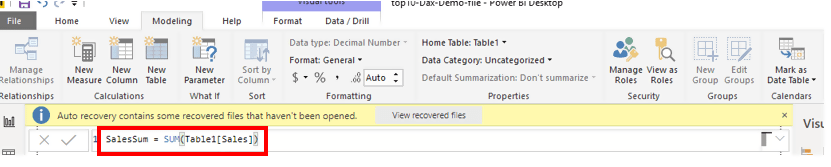Step 2: To check, use Card visualization and drag SalesSum on the canvas. We can see below that the total sales figure is just above 81 million.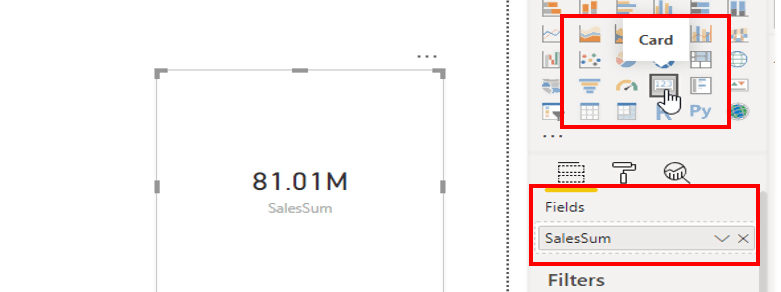## SUMX ()

Suppose you want to compute sales revenue by multiplying the order quantity by unit price. This means that you have to compute a column which calculates [unit price] * [order Qty] and then adds the result using SUM (). This 2-step process can be easily done in a single step using SUMX (). The syntax is given below

SUMX (Table, Expression)

In our example to calculate total sales, we use SUMX (shown below). Inside the bracket, table1 shows the table which will be evaluated. The expression after comma states the formula to be computed row by row.To check whether our SUMX measure is working drag Total Sales on the canvas using card visualization and it shows the total sales correctly.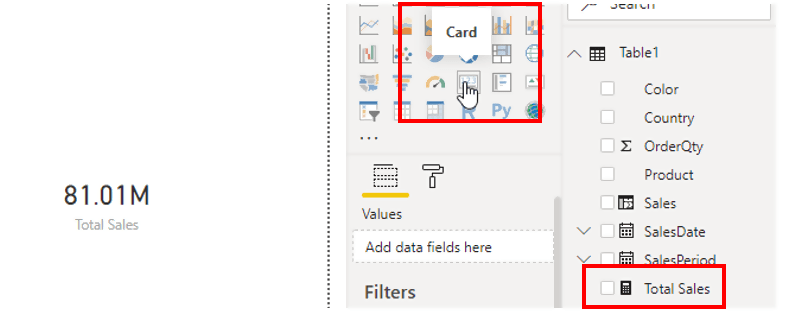## AVERAGE ()

The average function returns the average (arithmetic mean) of all the numbers in a column. Following is the syntax for Average ()

AVERAGE (Column Name)

For example, if we want to know the average sales for each product category following steps need be taken.

Step 1: Click on New Measure under the Modeling tab. Rename the measure as Sales Average. The syntax shown below will store a calculation that will perform an average on sales.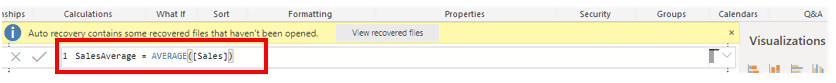Step 2: Select Table visualization and drop Product and SalesAverage in the table created and we will have the following table displaying averages of each product’s sales.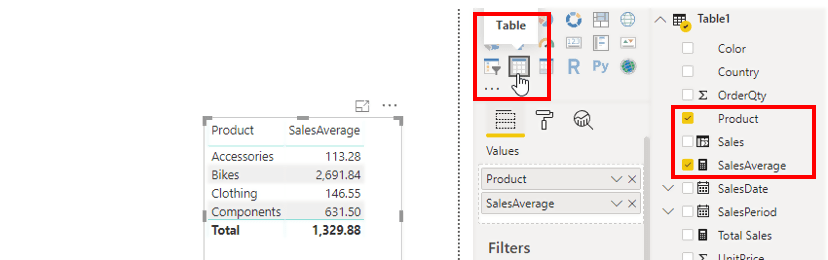## AVERAGEX()

Just like SUMX the AverageX computes the average of an expression. This saves extra steps of computing the total and then take the average.

The syntax for AVERAGEX is as follows

AVERAGEX (Table Name, Expression)

The following steps explain how to find the Average of sales revenue.

Step 1: Create a new measure as SaleAverageX. Write Table and expression inside AVERAGEX () as shown below.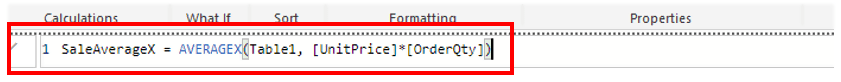Step 2: Select Table visualization and drop Product, Sales Average and Sales AverageX in the table created and we will have the following table displaying averages of each product’s overall sales. Here both AVERAGE and AVERAGEX seem to be doing the same thing, however, AverageX will be useful when sales values are not available, and we have to compute the sales value by multiplying order Qty by Price.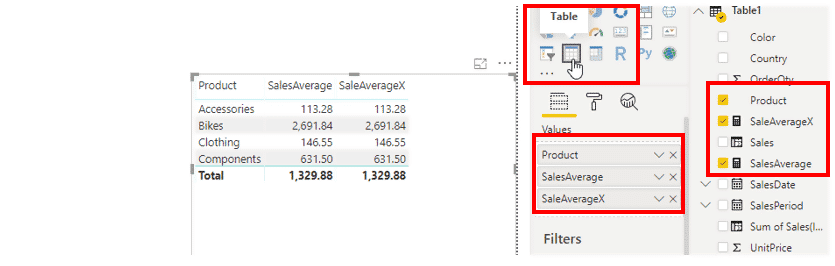## MI­­­­­N ()

The MIN function returns the smallest value in a column, or between two scalar expressions. The syntax for MIN is as follows.

MIN (Column Name) or MIN (expression1, Expression2)

Following steps guide how to use MIN function to find the minimum of sales column

Step 1: Create a new measure named as Minimum Sales as shown belowStep 2: Using Card Visualization drag Sales Column on the canvas. The card below will show the overall minimum sales of the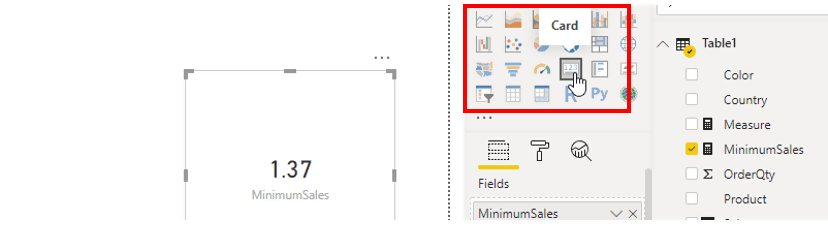Step 3: The user can also see the minimum sales for each product category by dragging product and Minimum sales (shown below)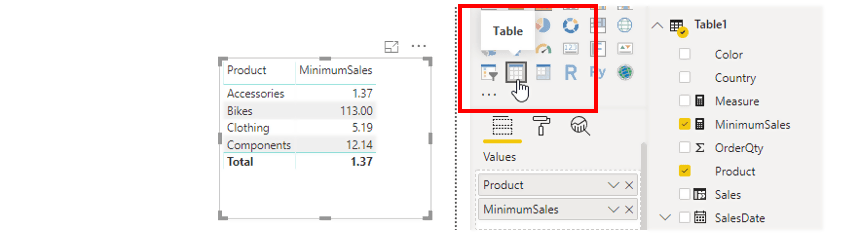## MAX()

In order to extract maximum value from a column, we use MAX function which is the opposite of MIN function i.e. it gives the maximum value in a column, or between two scalar expressions. The syntax for MAX function is

MAX(<column>) or MAX(<expression1>, <expression2>)

The following steps explain how to use MAX to find the maximum of Sales value by country

Step 1: Create a new measure named Maximum Sales using the Sales column inside MAX () function as shown below.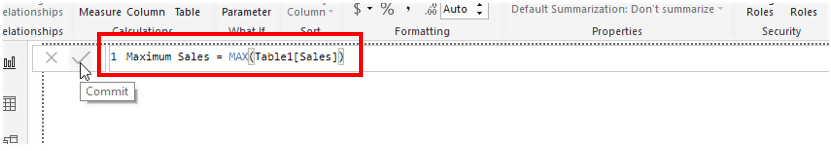Step 2: Using matrix visualization drag the columns of Country, Product and Maximum Sales in the Rows, Column and Values field as shown below. We can clearly see that the United States has experienced the highest sales in the category of Bikes.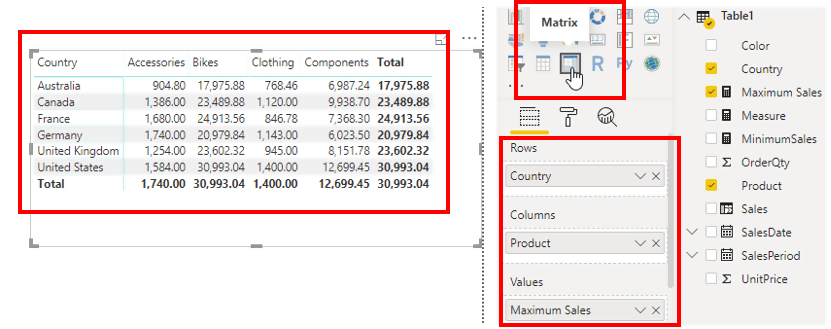## CALCULATE ()

The CALCULATE function is a very important function in Power BI as it is used to apply your own filters to data, that can add to existing filters or even replace them.

The following syntax is followed to write a CALCULATE function.

CALCULATE(<expression>, <filter1>, <filter2>…)

The step by step guide below demonstrates how we use Calculate to fund the SUM of sales where Sales are of products which are red in color.

Step 1: Click on New Measure under the Modeling tab.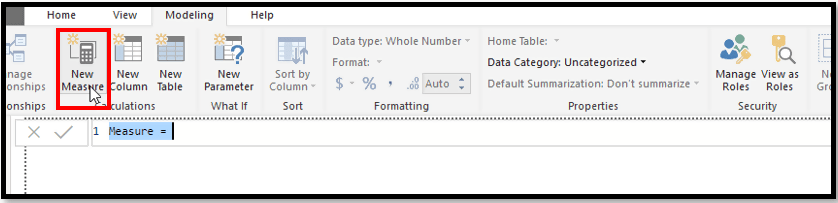Step 2: Rename the measure as Total Sales (Red). This measure will create a calculation that will filter the Red Sales out of the total Sales. The CALCULATE function will evaluate Table1 and column Total Sales based on Product Color as Red.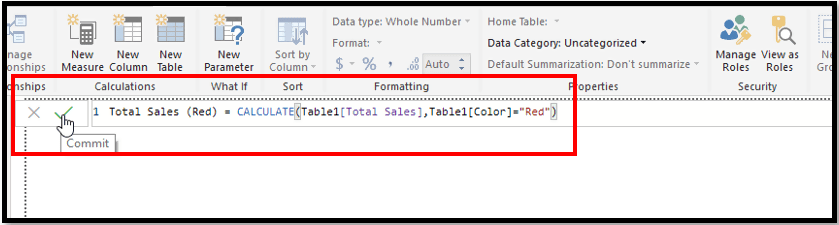Step 3: To check whether the new Total Sales Red measure shows red sales only, drag Country, Total Sales Red and Total Sales on the canvas. As shown below the Total Sales Red column shows sales of Products for each country which are Red only.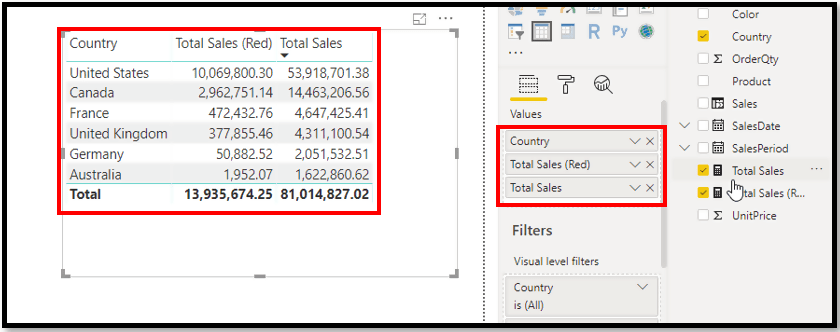Step 4 : The Calculate Function can also evaluate an expression for multiple filters as shown below. Here the function filters Sales which are both Red and for the United States Only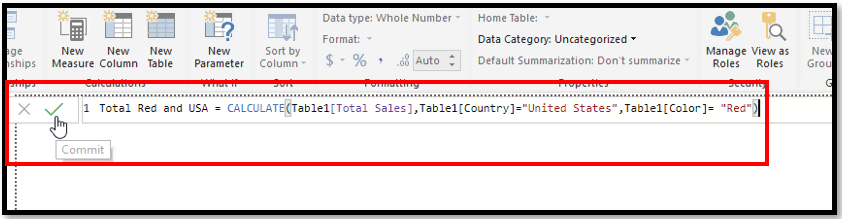## Filter ()

The filter function works the same way as CALCULATE; however, its major difference is that the FILTER functions are not mutable; It can only subset the data. FILTER is an expression that can be used in unison with an existing function such as CALCULATE the SUMX, we have used Filter to FILTER the unit price which is greater than 300 to calculate sales of the product which are expensive (greater than 300)

Following syntax is followed to write a Filter function

(<expression>,<filter1>,<filter2>…)

Step 1: Create a new measure and name it as Sales from expensive Goods. Here we will use an iterator Sumx which will perform a calculation of order Qty into Unit Price row by row. The Filter function will restrict the calculation of only those products with Unit Price greater than 300.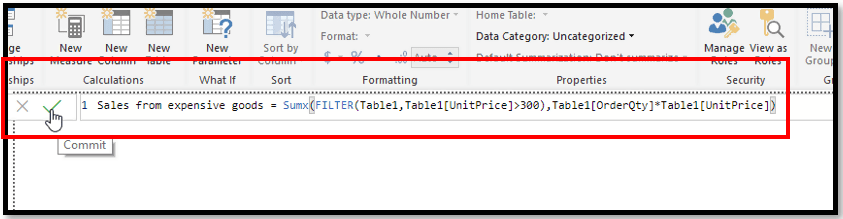Step 2: Drag and drop Product, Total Sales and Sales from expensive goods on the canvas. Here we can see that Sales from expensive goods (product with a unit price greater than 300) only belong to Bikes and Components and yet generate more than 90% of total sales.

## ALL Function

ALL function returns all the rows in a table or column, regardless of applied filters. Its simply ignores all filters and clears them. This function is very useful when we calculate aggregations, for s on all the rows in a table. This function is usually nested inside CALCULATE or AVERAGEX.

Syntax

ALL(<table>[<column>])

Example of ALL Function with Steps

Step 1:  We will use All function to calculate Total Sales which will ignore any filters. We will create a new measure named Sales Grand Total and we will use the CALCULATE function that will calculate the sum of Sales. However, the ALL function which will be clearer in the next steps will ignore any filters that will be applied when generating the reports.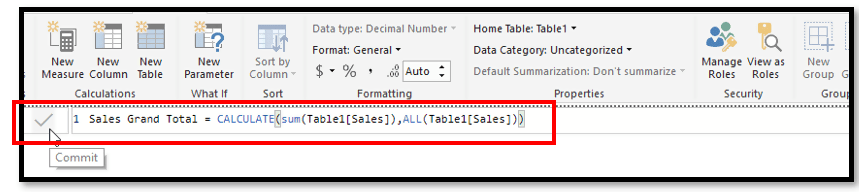Step 2: To demonstrate the point. We drag the Product, Sales and Sales Grand Total on the canvas. As we can see that Sales Grand Total doesn’t change for any filters. This is very useful when we want to calculate denominators for calculating percentages and ratios.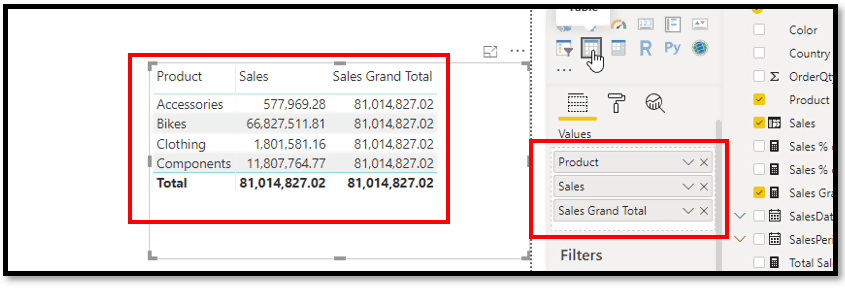## MINX ()/ MAXX ().

We have kept the MINX/MAXX function in the end as it utilizes all the DAX functions discussed above.  The MAXX function evaluates an expression for each row of a table and returns the largest value. Similarly, MINX function does the opposite i.e. returns the smallest value. Following is the syntax for MINX and MAXX function

MINX(<table>, < expression>)

MAXX(<table>, <expression>)

We will discuss the MAXX () function in our example below. Suppose you want to find the maximum value of a sales transaction of each product category for the color black only. Following steps should be taken

Step 1:  Create a measure named Max Black Sales. We will use CALCULATE () as an iterator i.e. it will search on the maximum of sales column row by row and find maximum sales of black color only.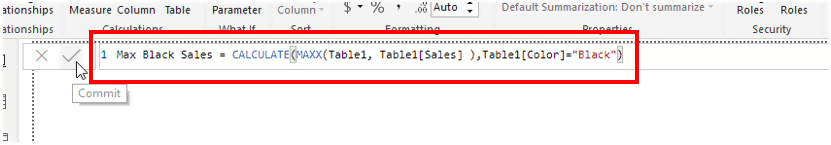Step 2:  Using Table Visualization drag Product and Max Black Sales on the canvas. We can clearly see that the maximum value of a sales transaction has incurred for the color black is in the Bikes category. Bikes are expensive!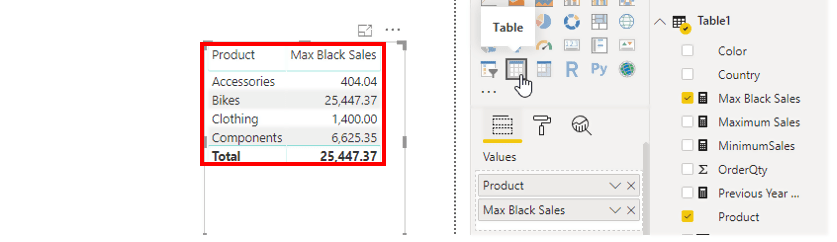#### Gaelim Holland

Subscribe
Notify ofInline Feedbacks